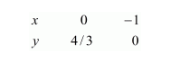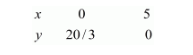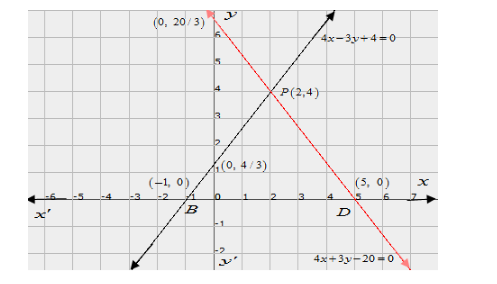# Solve graphically the system of linear equations:

Question:

Solve graphically the system of linear equations:

$4 x-3 y+4=0$

$4 x+3 y-20=0$

Find the area bounded by these lines and x-axis.

Solution:

The given equations are

$4 x-3 y+4=0$$. .(i) 4 x+3 y-20=0$$. .(i i)$

Putting $x=0$ in equation $(i)$, we get:

$\Rightarrow 4 \times 0-3 y=-4$

$\Rightarrow y=4 / 3$

$x=0, \quad y=4 / 3$

Putting $y=0$ in equation $(i,$, we get:

$\Rightarrow 4 x-3 \times 0=-4$

$\Rightarrow x=-1$

$x=-1, \quad y=0$

Use the following table to draw the graph.The graph of (i) can be obtained by plotting the points $(0,4 / 3),(-1,0)$.

$4 x+3 y=20$ ..(ii)

Putting $x=0$ in equation $(i i)$ we get:

$\Rightarrow 4 x+3 \times 0=20$

$\Rightarrow x=5$

$x=5, \quad y=0$

Use the following table to draw the graph.Draw the graph by plotting the two points from table.The two lines intersect at $P(2,4)$.

Hence $x=2, \quad y=4$ is the solution of the given equations.

Now,

$\Rightarrow$ Required area $=$ Area of PBD

$\Rightarrow$ Required area $=1 / 2($ base $\times$ height $)$

$\Rightarrow$ Required area $=1 / 2(B D \times P M)$

$\Rightarrow$ Required area $=1 / 2(6 \times 4)$

Hence, the area $=12$ sq.units